# Convert Column Letter to Number – Excel & Google Sheets

This tutorial demonstrates how to convert a column letter to number in Excel.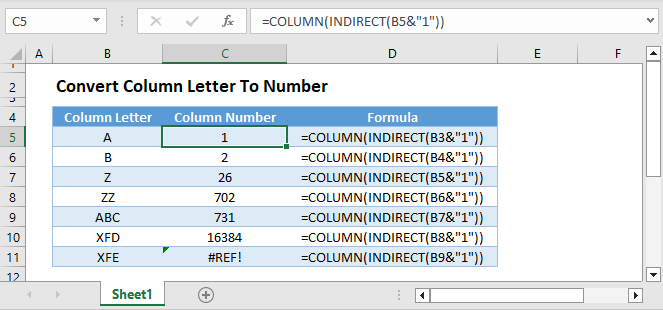## Converting Column Letter to Number

To get the number of a column letter in Excel, we will use the COLUMN and INDIRECT Functions.

``=COLUMN(INDIRECT(B3&1))``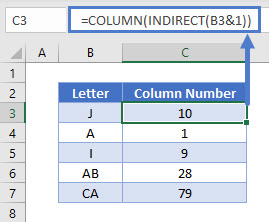## INDIRECT Function

The INDIRECT Function converts a string of text corresponding to a cell reference, into the actual cell reference.

``=INDIRECT("A1")``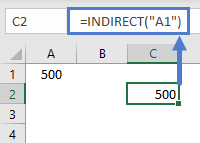## COLUMN Function

The cell reference is then passed to the COLUMN Function, which returns the number of the column.

``=COLUMN(Cell Reference)``

To illustrate, let’s see some examples below.

=COLUMN(A1) has a result of 1.  This is because the cell reference “A1” is in column number 1.

=COLUMN(B1) has a result of 2.  This is because the cell reference “B1” is in column number 2.

=COLUMN(H1) has a result of 8.  This is because the cell reference “H1” is in column number 8.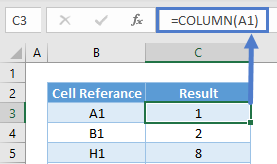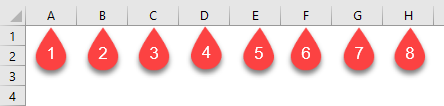## Convert Column Letter to Number in Google Sheets

The combination of COLUMN and INDIRECT Functions works exactly the same in Google Sheets as in Excel: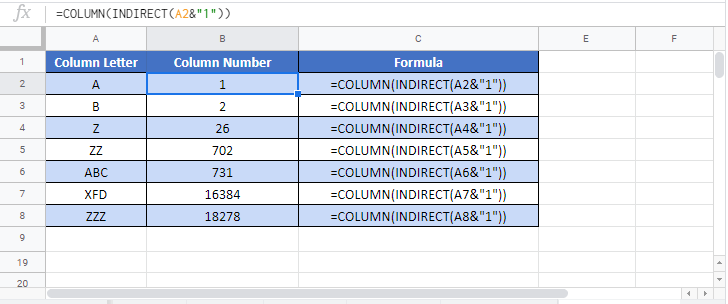### Excel Practice Worksheet

Practice Excel functions and formulas with our 100% free practice worksheets!